﻿ FN8411

Specification

Practice Test

# Number Sets

Number Sets are categories of number types

### Natural

Positive Integers (whole numbers)
1, 2, 3, 4, 5 etc.

### Integer

This includes Natural numbers and their negatives

-5, -4, -3, -2, -1, 0, 1, 2, 3, 4, 5

### Rational

Rational numbers are the ratios of integers, also called fractions, such as 1/2 = 0.5 or 1/3 = 0.333…

### Real

Real numbers include non-whole numbers, for example, 2.13, 8.4656, 9,102.3

Real numbers include transcendental numbers, that is, numbers with infinite description, such as pi

# Decimal to Binary

Everything inside computers is made up of binary. Binary just means one of two. This can be True or False. Yes or No, On or Off. 1 or 0.

We need to know how to figure out what some numbers are in binary and turn them into normal numbers that normal people use. (and the other way round).

This is 8 bit binary btw, It’s the only type you have to worry about. It means the biggest number you will be asked about is 255. Anything else is probably a trick question :S

So, 25 in binary is 11001

Why?

Don’t know, but I’ll show you how to figure it out tl:dr…

So, you basically take your number

25

divide it in two

12.5 and dingy the .whatever so 12

divide it in two

6

divide it in two

3

divide it in two

1.5, dingy the .whatever again, so 1

so we have 1, 3, 6, 12, 25

For each number now we see if it is odd or even, odd gets 1, even gets 0, so…

1 1 0 0 1

Bosh, we got our answer, and we don’t even know what’s going on!

Done!

# Hexidecimal to DecimalWhen taking a Hexadecimal number, for three figure numbers, we use the equation

(Character1 * 256) + (Character2 * 16) + (Charater3)

For Example

6FF = (6 * 256) + (15 * 16) + (15)

or 1,536 + 240 + 15 = 1,791

This equation is a simplified version of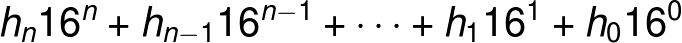Where you can substitute 16 for which ever base you with to use.

# Interior AnglesInterior Angles of a triangle will all add up to 180 Degrees.

For most simple shapes we can break them down into multiple triangles.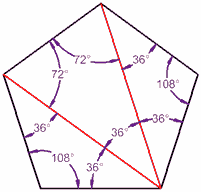By using this method we can ascertain the interior angles of simple 2D shapes.

a + b + c = 180°

To find the total interior angle of a shape we can use the equation ( n- 2 ) 180°, where n is the number of sides

# Areas and Perimeters

The area of regular four side shapes can be calculated by multiplying the length and height together.2 x 4 = 8²

The area of a circle can be calculated using πr²

π ( pi ) can is an infinite number but can be rounded off to two decimal places for these calculations, 3.14π2² = 12.57

# Volumes and Surface Area

The surface area of a cube can be calculated by multiplying the area by the depth

4² x 2 = 8³

# Vectors

A vector describes a movement from one point to another. A vector quantity has both direction and magnitude (size).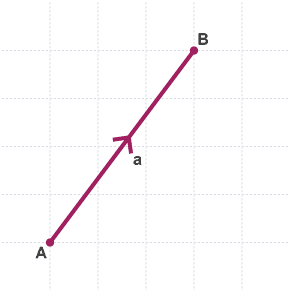The Vector below, represents move across 3 units to the right, and, then down by 2 units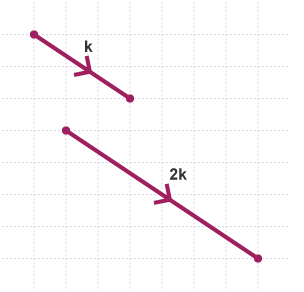When we multiply a a vector by a scalar, we simply multiply each number# Simplifying Equations

BODMAS is a useful acronym that lets you know which order to solve mathematical problems.

The BODMAS acronym is for:

• Brackets (parts of a calculation inside brackets always come first).
• Orders (numbers involving powers or square roots).
• Division.
• Multiplication.
• Subtraction.

# Random Number Generators

Random number generators are usually called with a function RND
In order to receive an expected output we need to define the seed value.
The see value defines the maximum number output from the random number generator.
It is common to manipulate Random Number Generators to conform to human expectation, as, we commonly reject or see patterns in truly random sequences. ie, we may see a single number repeated

# Expressing negative Indicies

Indicies are practically powers of
We can express negative powers in order to generate a negative output. This can also be a more efficient way to store negative numbers.

# Flow Charts

Flow charts express how the logic of a sequence of events plays out. By using the below key we can express this graphically, allowing us to plan events before programming.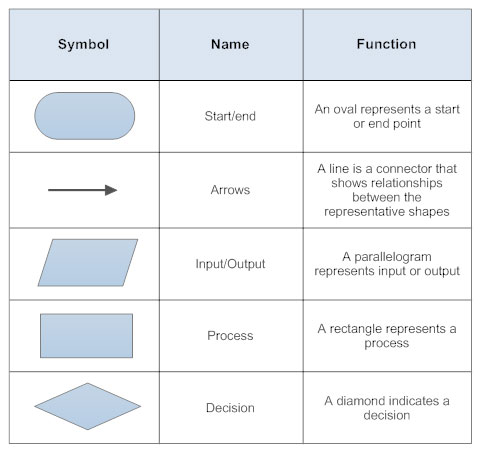flow.io